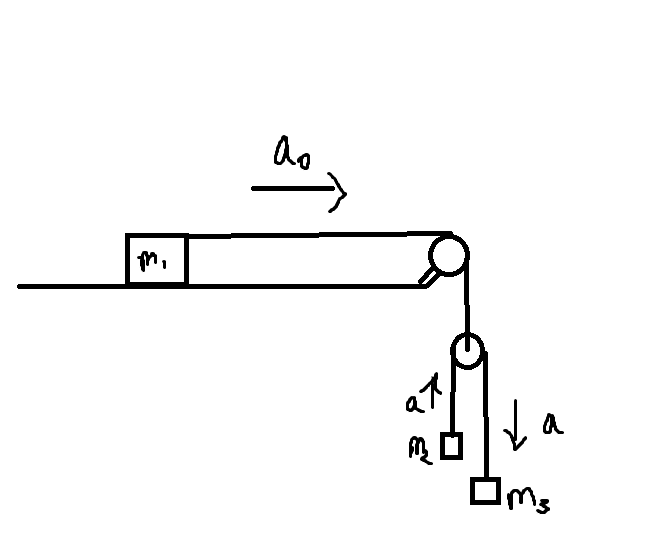# Pseudo forces and negative acceleration

• EddiePhys
Yes! If a and a0 are in the same direction, and we assumed that they are opposite in direction one of them would come out to be negative and correct us. Just put in the signs of the forces correctly.

## Homework StatementF = ma

## The Attempt at a Solution

[/B]
I already have the solution, however, I have a few questions.
1)In the solution they have taken m2's acceleration relative to the ground to be a0-a downwards.
However, if a>a0, then wouldn't the acceleration relative to the ground end up being being upwards? In that case, how is assuming the acceleration downwards and being equal to a0-a correct?

2) If viewed from the accelerating frame of the movable pulley,

pseudo force upwards = m2a0
therefore, the eq of motion for m2: T-m2g+m2a0 = m2a
=> T-m2g = m2(a-a0)

Why does the pseudo force method lead to an answer that is "biased" towards m2 accelerating upwards relative to the ground? i.e assuming m2 will be accelerating upwards from the ground frame?

Here we have just assumed a>a0. If our assumption is wrong, the acceleration will become negative and tell us that our assumption is opposite to what actually is happening. You can assume m3 to be accelerating upwards, you will get the same answer but with different signs.

AbhinavJ said:
Here we have just assumed a>a0. If our assumption is wrong, the acceleration will become negative and tell us that our assumption is opposite to what actually is happening. You can assume m3 to be accelerating upwards, you will get the same answer but with different signs.

The answer in the end comes up to a0 = g/(1+m1/4(1/m2 + 1/m3)). Since a has been eliminated from the equation, would this still be right if a>a0?

Last edited:
EddiePhys said:
The answer in the end comes up to a0 = g/(1+m1/4(1/m2 + 1/m3)). Since a has been eliminated from the equation, would this still be right if a>a0?
Yes! If a and a0 are in the same direction, and we assumed that they are opposite in direction one of them would come out to be negative and correct us. Just put in the signs of the forces correctly.